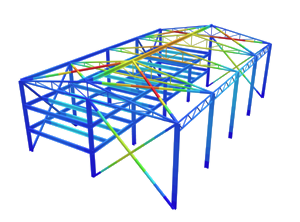SkyCiv Documentation

Your guide to SkyCiv software - tutorials, how-to guides and technical articles

1. Home
2. Tutorials
3. Truss Tutorials
4. Tutorial to Solve Truss by Method of Sections

# Tutorial to Solve Truss by Method of Sections

## Tutorial: How to Solve a Truss Structure using Method of Sections

In this tutorial, we will explore and learn the benefits of using the Method of Sections to solve your Truss Structure. What are trusses? If you’re unsure about this, visit our What is a truss tutorial. The method of Sections is used to solve larger truss structures in a fast, simple manner. It involves taking a ‘cut’ through a number of members to evaluate their axial forces and use this as our basis to solve the rest of the truss structure. So first, let’s look at an example question:

#### Question: Using the method of sections, determine the forces in members 10, 11, and 13 of the following truss structure: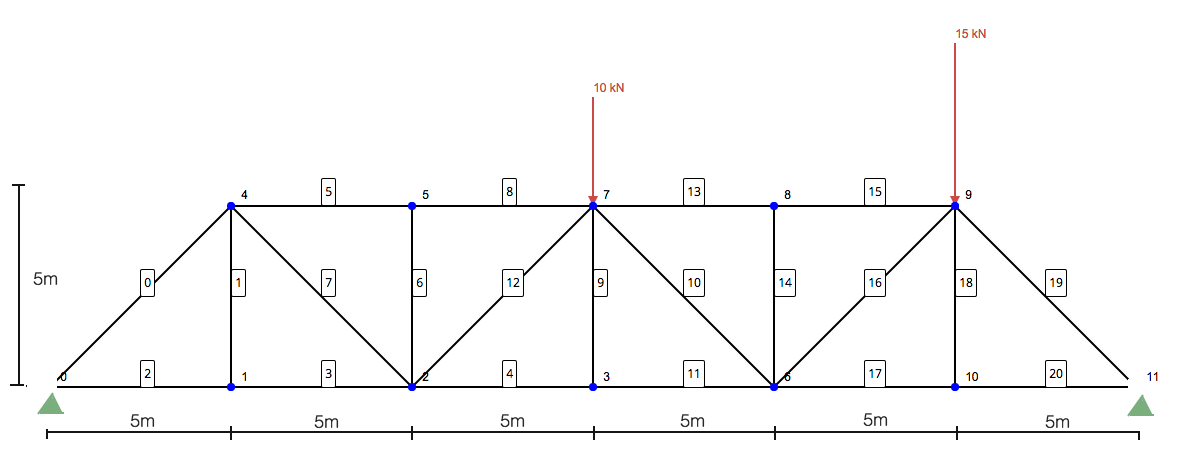## Step 1: Calculate the Reactions at the Supports

Like most static structural analysis, we must first start by locating and solving the reactions at supports. This will give us the boundary conditions we need to progress in solving the truss structure. Simplifying the structure to just include the loads and supports: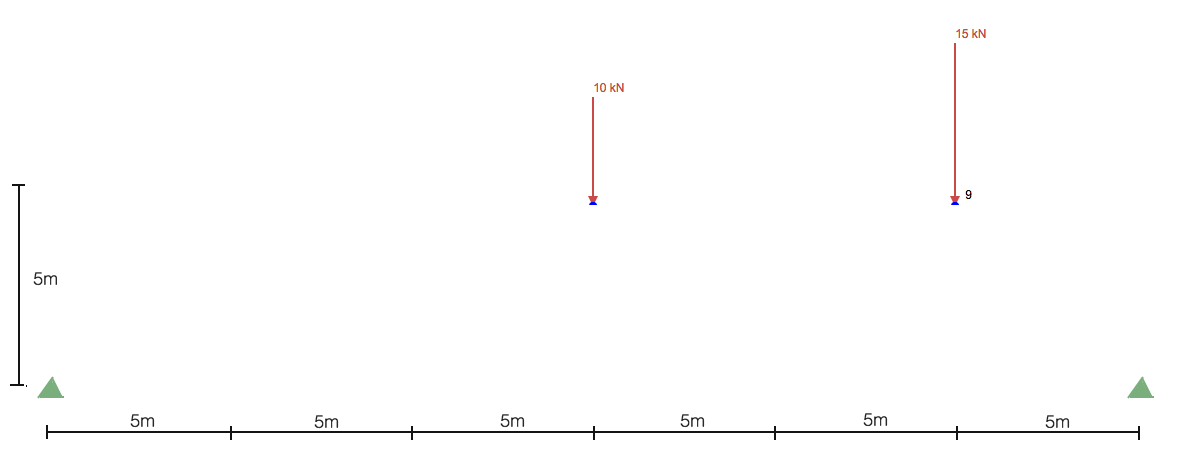Without spending too much time calculating the reactions, you generally start by taking the sum of moments about a point. Taking the sum of moments about the left support gets us: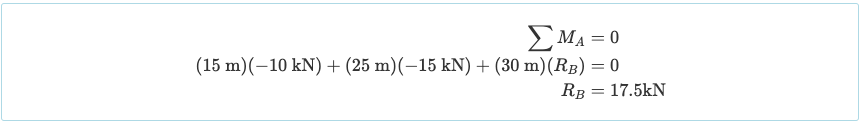So the reaction at the right support (RB) is 17.5 kN in an upward direction. Now, taking the sum of forces in the y gives us the reaction RA as 7.5kN in an upward direction: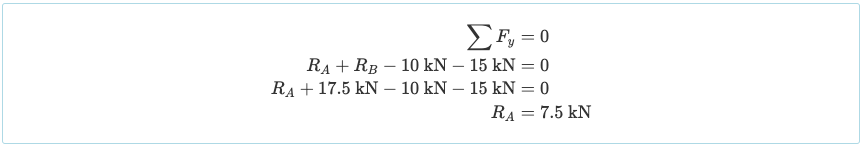## Step 2: Make a cut along the members of interest

Here comes the most important part of solving a truss using the method of Sections. It involves making a slice through the members you wish to solve. This method of structural analysis is extremely useful when trying to solve some of the members without having to solve the entire structure using the method of joints. So, in our example here would be our slice: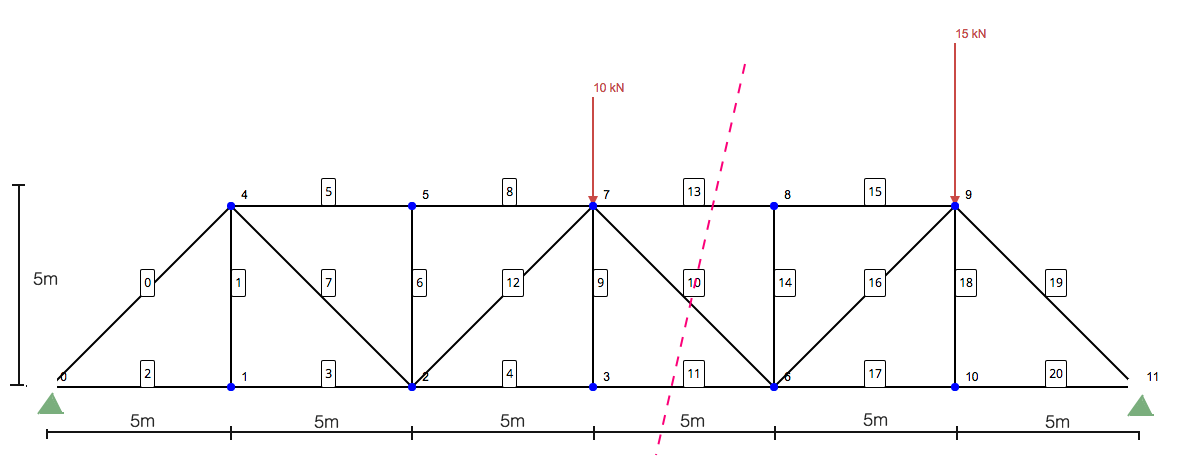Focussing on the left side only, you are left with the following structure: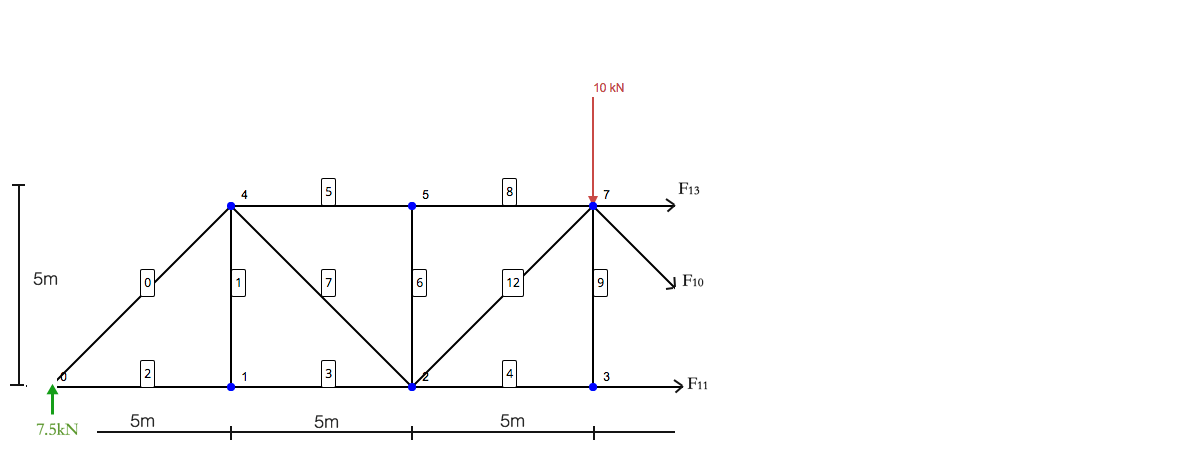Now think of this structure as single standing structure. The laws of statics still apply – so the sum of moments and forces must all equal zero. The members with arrows (F13, F10, F11) are what stabilize the reaction and forces applied to the structure. Note that the sum of moments is taken about node 7 – as would exclude the forces of members 13 and 10 – leaving F11 to be isolated.

Using the above Free Body Diagram, we can obtain the following formulae:

Sum of forces in the y-direction: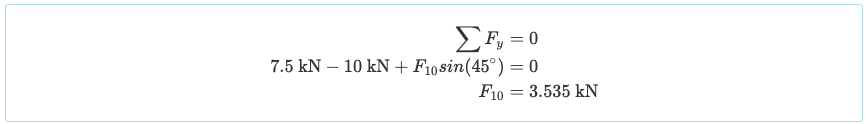Sum of moments about node 7: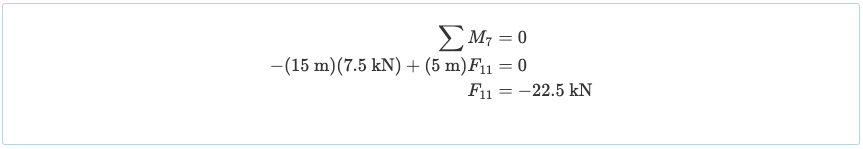Sum of forces in the x-direction: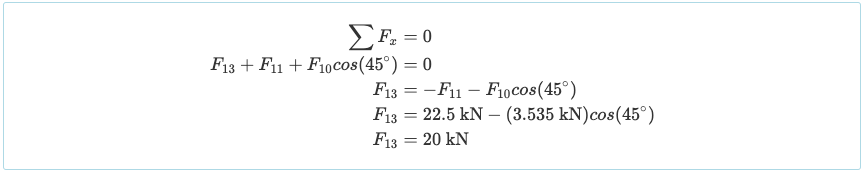## Final Solution

We can use these results to solve the remaining members in the truss structure. We hope this example has been useful and feel free to comment with your questions below. As a reference, the results for the entire Truss structure can be found below (using our Truss Calculator) which is great for checking your answers!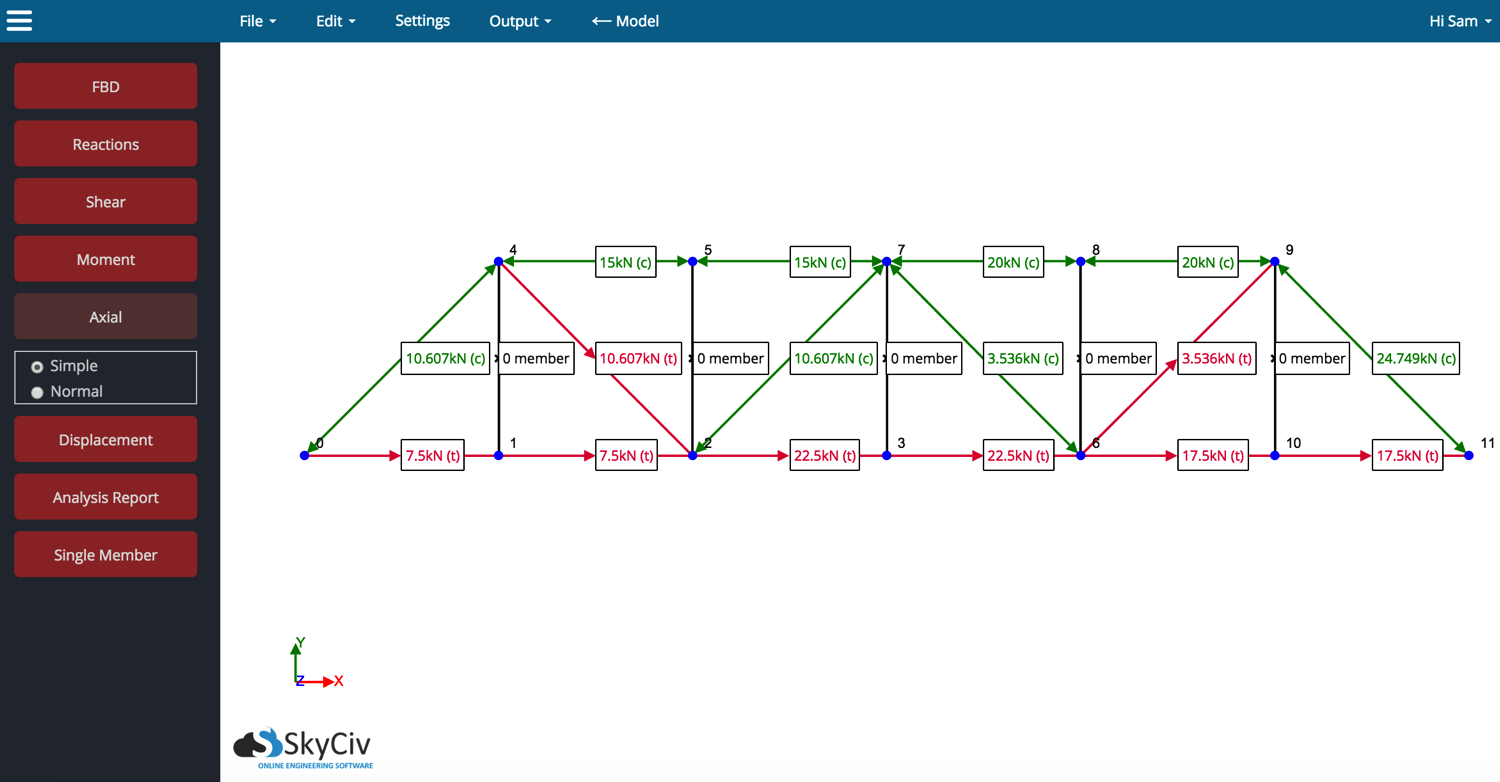## Simple Steps

• Always Start by calculating reactions at supports
• Make a slice through the members you wish to solve
• Treat the half structure as its own static truss
• Solve the truss by taking the sum of forces = 0
• Take the moment about a node of more than one unknown member